# Common Core: Kindergarten Math : Counting the Number of Objects

## Example Questions

← Previous 1 3 4 5 6 7 8 9 22 23

### Example Question #1 : Know Number Names In Order And Pair Objects With A Number Name: Ccss.Math.Content.K.Cc.B.4a

Select the answer with two triangles.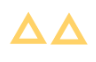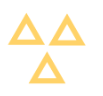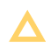Explanation:

The numberas a word is two.### Example Question #2 : Know Number Names In Order And Pair Objects With A Number Name: Ccss.Math.Content.K.Cc.B.4a

Select the answer with three triangles.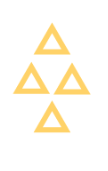Explanation:

The numberas a word is three.### Example Question #3 : Know Number Names In Order And Pair Objects With A Number Name: Ccss.Math.Content.K.Cc.B.4a

Select the answer with four triangles.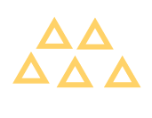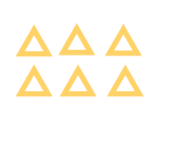Explanation:

The numberas a word is four.### Example Question #4 : Know Number Names In Order And Pair Objects With A Number Name: Ccss.Math.Content.K.Cc.B.4a

Select the answer with five triangles.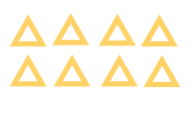Explanation:

The numberas a word is five.### Example Question #5 : Know Number Names In Order And Pair Objects With A Number Name: Ccss.Math.Content.K.Cc.B.4a

Select the answer with six triangles.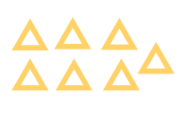Explanation:

The numberas a word is six.### Example Question #6 : Know Number Names In Order And Pair Objects With A Number Name: Ccss.Math.Content.K.Cc.B.4a

Select the answer with seven triangles.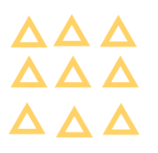Explanation:

The numberas a word is seven.### Example Question #7 : Know Number Names In Order And Pair Objects With A Number Name: Ccss.Math.Content.K.Cc.B.4a

Select the answer with eight triangles.Explanation:

The numberas a word is eight.### Example Question #8 : Know Number Names In Order And Pair Objects With A Number Name: Ccss.Math.Content.K.Cc.B.4a

Select the answer with nine triangles.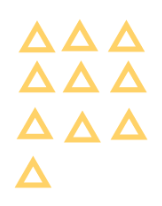Explanation:

The numberas a word is nine.### Example Question #1 : Counting The Number Of Objects

Select the answer with ten triangles.Explanation:

The numberas a word is ten.

### Example Question #1 : Counting The Number Of Objects

Select the answer with eleven triangles.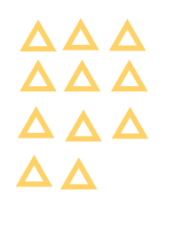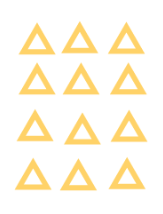Explanation:

The numberas a word is eleven.← Previous 1 3 4 5 6 7 8 9 22 23

### All Common Core: Kindergarten Math Resources